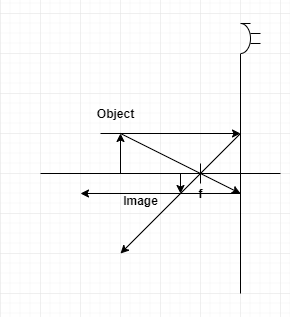# Problem: A light bulb is 60cm from a concave mirror with a focal length of 20cm. Use ray tracing to determine the location of its image. Is the image upright or inverted? Is it real or virtual? Verify your results by doing the calculations.

###### FREE Expert Solution

Mirror equation,

$\overline{)\frac{\mathbf{1}}{\mathbf{f}}{\mathbf{=}}\frac{\mathbf{1}}{{\mathbf{s}}_{\mathbf{i}}}{\mathbf{+}}\frac{\mathbf{1}}{{\mathbf{s}}_{\mathbf{o}}}}$

A ray parallel to the principal axis is reflected through f.

A ray through f is reflected parallel to the principal axis.###### Problem Details

A light bulb is 60cm from a concave mirror with a focal length of 20cm. Use ray tracing to determine the location of its image. Is the image upright or inverted? Is it real or virtual? Verify your results by doing the calculations.